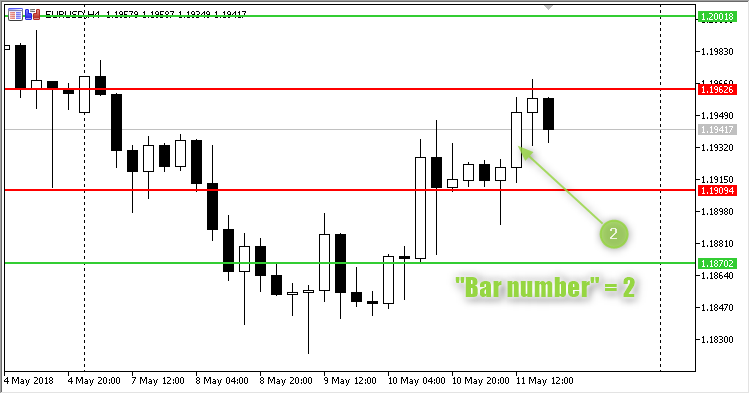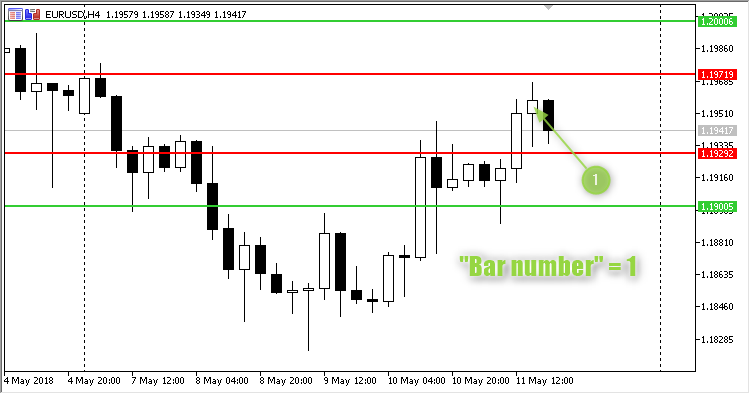# Four horizontal lines – indicator for MetaTrader 5

• A+
category：MT5 INDICATORS

The indicator plots four horizontal lines: Two basic ones (red by default) above the High and below the Low of the Bar number bar and help lines (green by default) shifted from the basic ones.

### Basic lines plotting rule

• Line HighLowUP is plotted by the High of the Bar number plus Shift;
• Line HighLowDOWN is plotted by the Low of the Bar number minus Shift.

### Help lines plotting rule

• Line DifferenceUP is plotted by the HighLowUP price PLUS the difference between prices HighLowUP and HighLowDOWN and MINUS Shift.

• Line DifferenceDOWN is plotted by the HighLowDOWN price MINUS the difference between prices HighLowUP and HighLowDOWN and PLUS Shift.

This indicator is mostly used on large timeframes, starting from H4.Fig. 1. Parameter "Bar number" is "2"Fig. 2. Parameter "Bar number" is "1"